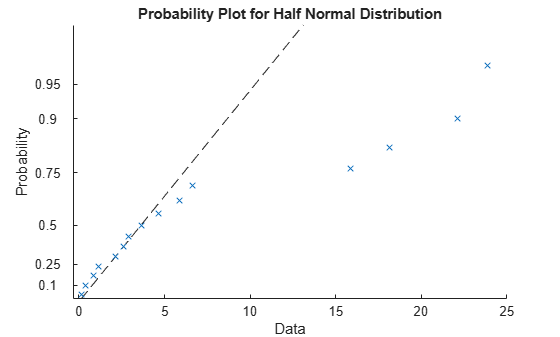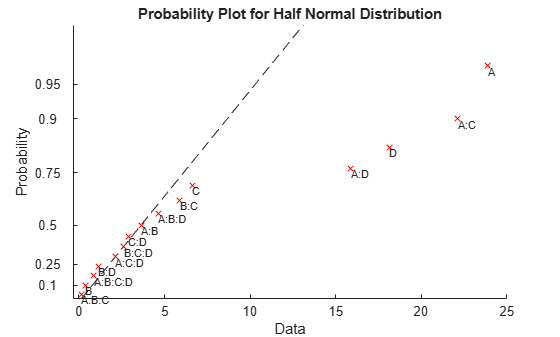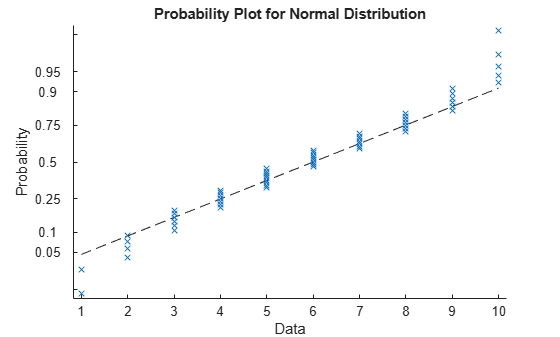Documentation

This is machine translation

Mouseover text to see original. Click the button below to return to the English version of the page.

probplot

Probability plots

Syntax

probplot(y)
probplot(y,cens)
probplot(y,cens,freq)
probplot(dist,___)
probplot(ax,___)
probplot(ax,pd)
probplot(ax,fun,params)
probplot(___,'noref')
h = probplot(___)

Description

example

probplot(y) creates a normal probability plot comparing the distribution of the data in y to the normal distribution.probplot plots each data point in y using marker symbols and draws a reference line that represents the theoretical distribution. If the sample data has a normal distribution, then the data points appear along the reference line. The reference line connects the first and third quartiles of the data and extends to the ends of the data. A distribution other than normal introduces curvature in the data plot.
probplot(y,cens) creates a probability plot using the censoring data in cens.

example

probplot(y,cens,freq) creates a probability plot using the censoring data in cens and the frequency data in freq.

example

probplot(dist,___) creates a probability plot for the distribution specified by dist, using any of the input arguments in the previous syntaxes.
probplot(ax,___) adds a probability plot into the existing probability plot axes specified by ax, using any of the input arguments in the previous syntaxes.
probplot(ax,pd) adds a fitted line on the existing probability plot axes specified by ax to represent the probability distribution pd.

example

probplot(ax,fun,params) adds a fitted line on the existing probability plot axes specified by ax to represent the function fun with the parameters params.
probplot(___,'noref') omits the reference line from the plot.

example

h = probplot(___) returns graphics handles corresponding to the plotted lines.

Examples

collapse all

Generate sample data and create a probability plot.

Generate sample data. The sample x1 contains 500 random numbers from a Weibull distribution with scale parameter A = 3 and shape parameter B = 3. The sample x2 contains 500 random numbers from a Rayleigh distribution with scale parameter B = 3.

rng('default'); % For reproducibility x1 = wblrnd(3,3,[500,1]); x2 = raylrnd(3,[500,1]);

Create a probability plot to assess whether the data in x1 and x2 comes from a Weibull distribution.

figure probplot('weibull',[x1 x2]) legend('Weibull Sample','Rayleigh Sample','Location','best')The probability plot shows that the data in x1 comes from a Weibull distribution, while the data in x2 does not.

Alternatively, you can use wblplot to create a Weibull probability plot.

Create a probability plot and an additional fitted line on the same figure.

Generate sample data containing about 20% outliers in the tails. The left tail of the sample data contains 10 values randomly generated from an exponential distribution with parameter mu = 1. The right tail contains 10 values randomly generated from an exponential distribution with parameter mu = 5. The center of the sample data contains 80 values randomly generated from a standard normal distribution.

rng('default') % For reproducibility left_tail = -exprnd(1,10,1); right_tail = exprnd(5,10,1); center = randn(80,1); data = [left_tail;center;right_tail];

Create a probability plot to assess whether the sample data comes from a normal distribution.

probplot(data)

Plot a t location-scale curve on the same figure to compare with data.

p = mle(data,'distribution','tLocationScale'); t = @(data,mu,sig,df)cdf('tLocationScale',data,mu,sig,df); h = probplot(gca,t,p); h.Color = 'r'; h.LineStyle = '-'; title('{\bf Probability Plot}') legend('Normal','Data','t','Location','NW')The plot shows that neither the normal line nor the t location-scale curve fits the tails very well because of the outliers.

Create a half-normal probability distribution plot to identify significant effects in an experiment to study factors that might influence flow rate in a chemical manufacturing process. The four factors are reactants A, B, C, and D. Each factor is present at two levels (high and low concentration). The experiment contains only one replication at each factor level.

load flowrate

The first four columns of the table flowrate contain the design matrix for the factors and their interactions. The design matrix is coded to use 1 for the high factor level and -1 for the low factor level. The fifth column of flowrate contains the measured flow rate.

Fit a linear regression model using rate as the response variable. Use predictor variables A, B, C, D, and all of their interaction terms.

mdl = fitlm(flowrate,'rate ~ A*B*C*D');

Calculate and store the absolute value of the factor effect estimates. To obtain the factor effect estimates, multiply the coefficient estimates obtained during the model fitting by two. This step is necessary because the regression coefficients measure the effect of a one-unit change in x on the mean of y. However, the effects estimates measure a two-unit change in x due to the design matrix coding of -1 and 1. Exclude the baseline measurement. Note that the factor order in mdl may be different from the order in the original design matrix.

effects = abs(mdl.Coefficients{2:end,1}*2);

Create a half-normal probability plot using the absolute value of the effects estimates, excluding the baseline.

figure h = probplot('halfnormal',effects);Label the points and format the plot. First, return the index values for the sorted effects estimates (from lowest to highest). Then use these index values to sort the probability values stored in the graphics handle (h(1).YData).

[b,i] = sort(effects); prob(i) = h(1).YData;

Add text labels to the plot at each point. For each point, the x-value is the effects estimate and the y-value is the corresponding probability.

text(effects,prob,mdl.CoefficientNames(2:end),'FontSize',8,... 'VerticalAlignment','top') h(1).Color = 'r';The points located far from the reference line represent the significant effects.

Generate simulated frequency data.

y = 1:10; freq = [2 4 6 7 9 8 7 7 6 5];

Create a normal probability plot using the frequency data.

probplot(y,[],freq)The normal probability plot shows that the data do not have a normal distribution.

Input Arguments

collapse all

Sample data, specified as a numeric vector or numeric matrix. probplot displays each value in y using marker symbols including 'x' and 'o'. If y is a matrix, then probplot displays a separate line for each column of y.

Not all distributions are appropriate for all data sets. probplot errors if the data set is inappropriate for a specified distribution. See dist for appropriate data ranges for each distribution.

Distribution for probability plot, specified as a probability distribution object or one of the following distribution names:

NamePlot TypeData Range
'normal'Normal probability plotAll values
'exponential'Exponential probability plotNonnegative values
'extreme value'Extreme value probability plotAll values
'half normal'Half-normal probability plotAll values
'lognormal'Lognormal probability plotPositive values
'logistic'Logistic probability plotAll values
'loglogistic'Loglogistic probability plotPositive values
'rayleigh'Rayleigh probability plotPositive values
'weibull'Weibull probability plotPositive values

The default is 'normal' if you create a probability plot in a new figure. If you add a probability plot to a figure that already includes one by using the ax input argument, then the default is the plot type of the existing probability plot.

You can create a probability distribution object with specified parameter values using makedist. Alternatively, fit a probability distribution object to sample data using fitdist. For more information on probability distribution objects, see Working with Probability Distributions.

The y-axis scale is based on the selected distribution. The x-axis has a log scale for the Weibull, loglogistic, and lognormal distributions, and a linear scale for the others.

Not all distributions are appropriate for all data sets. probplot errors if the data set is inappropriate for a specified distribution.

Example: 'weibull'

Censoring data, specified as a numeric vector. cens must be the same length as y, and contain a 1 value for observations that are right-censored and a 0 value for observations that are measured exactly.

Data Types: single | double

Frequency data, specified as a vector of integer values. freq must be the same length as y. freq contains the integer frequencies for the corresponding elements in y.

To create a probability plot using frequency data but not censoring data, specify empty brackets ([]) for cens.

Data Types: single | double

Target axes, specified as an Axes object or a UIAxes object. probplot adds an additional plot into the axes specified by ax. For details, see Axes Properties and UIAxes Properties.

Use gca to return the current axes for the current figure.

Probability distribution for reference line, specified as a probability distribution object. probplot adds a fitted line to the axes specified by ax to represent the probability distribution specified by pd.

Create a probability distribution object with specified parameter values using makedist. Alternatively, fit a probability distribution object to sample data using fitdist. For more information on probability distribution objects, see Working with Probability Distributions.

Function for reference line, specified as a function handle. probplot adds a fitted line to the axes specified by ax to represent the function specified by fun, evaluated at the parameters specified by params.

fun is a function handle to a cdf function, specified using the function handle operator @. The function must accept a vector of input values as its first argument, and return a vector containing the cdf evaluated at each input value. Specify the parameter values required to evaluate fun using the params argument. For more information on function handles, see Create Function Handle (MATLAB).

Example: @wblpdf

Data Types: function_handle

Reference line function parameters, specified as a vector of numeric values or a cell array. probplot adds a fitted line to the axes specified by ax to represent the function specified by fun, evaluated at the parameters specified by params.

fun is a function handle to a cdf function, specified using the function handle operator @. The function must accept a vector of values as its first argument, and return a vector of cdf values evaluated at each value. Specify the parameter values required to evaluate fun using the params argument. For more information on function handles, see Create Function Handle (MATLAB).

Output Arguments

collapse all

Graphic handles for line objects, returned as a vector of Line graphic handles. Graphic handles are unique identifiers that you can use to query and modify the properties of a specific line on the plot. For each column of y, probplot returns two handles:

• The line representing the data points. probplot represents each data point in y using marker symbols such as '+' and 'o'.

• The line showing the theoretical distribution for the probability plot, represented as a dashed line.

To view and set properties of line objects, use dot notation. For information on using dot notation, see Access Property Values (MATLAB). For information on the Line properties that you can set, see Line Properties.

Algorithms

probplot matches the quantiles of sample data to the quantiles of a given probability distribution. The sample data is sorted, scaled according to the choice of dist, and plotted on the x-axis. When dist is 'lognormal', 'loglogistic', or 'weibull', the scaling is logarithmic. Otherwise, the scaling is linear. The y-axis represents the quantiles of the distribution specified in dist, converted into probability values. The scaling depends on the given distribution and is not linear.

Where the x-axis value is the ith sorted value from a sample of size N, the y-axis value is the midpoint between evaluation points of the empirical cumulative distribution function of the data. In the case of uncensored data, the midpoint is equal to $\frac{\left(i-0.5\right)}{N}$.

probplot superimposes a reference line to assess the linearity of the plot. If the data is uncensored, then the line goes through the first and third quartiles of the data. If the data is censored, then the line shifts accordingly. If the data is uncensored and dist is 'half normal', then probplot uses the zeroth and second quartiles instead.